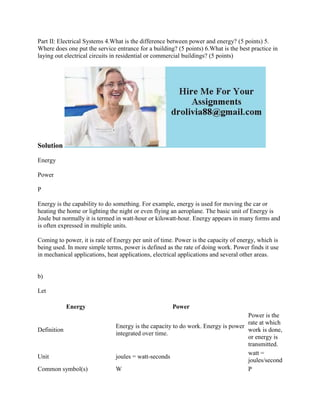Seu SlideShare está sendo baixado. ×

# Part II- Electrical Systems 4-What is the difference between power and.docx

Anúncio
Anúncio
Anúncio
Anúncio
Anúncio
Anúncio
Anúncio
Anúncio
Anúncio
Anúncio
AnúncioCarregando em…3
×

1 de 2 Anúncio

# Part II- Electrical Systems 4-What is the difference between power and.docx

Part II: Electrical Systems 4.What is the difference between power and energy? (5 points) 5. Where does one put the service entrance for a building? (5 points) 6.What is the best practice in laying out electrical circuits in residential or commercial buildings? (5 points)
Solution
Energy
Power
P
Energy is the capability to do something. For example, energy is used for moving the car or heating the home or lighting the night or even flying an aeroplane. The basic unit of Energy is Joule but normally it is termed in watt-hour or kilowatt-hour. Energy appears in many forms and is often expressed in multiple units.
Coming to power, it is rate of Energy per unit of time. Power is the capacity of energy, which is being used. In more simple terms, power is defined as the rate of doing work. Power finds it use in mechanical applications, heat applications, electrical applications and several other areas.
b)
Let

.

Part II: Electrical Systems 4.What is the difference between power and energy? (5 points) 5. Where does one put the service entrance for a building? (5 points) 6.What is the best practice in laying out electrical circuits in residential or commercial buildings? (5 points)
Solution
Energy
Power
P
Energy is the capability to do something. For example, energy is used for moving the car or heating the home or lighting the night or even flying an aeroplane. The basic unit of Energy is Joule but normally it is termed in watt-hour or kilowatt-hour. Energy appears in many forms and is often expressed in multiple units.
Coming to power, it is rate of Energy per unit of time. Power is the capacity of energy, which is being used. In more simple terms, power is defined as the rate of doing work. Power finds it use in mechanical applications, heat applications, electrical applications and several other areas.
b)
Let

.

Anúncio
Anúncio

### Part II- Electrical Systems 4-What is the difference between power and.docx

1. 1. Part II: Electrical Systems 4.What is the difference between power and energy? (5 points) 5. Where does one put the service entrance for a building? (5 points) 6.What is the best practice in laying out electrical circuits in residential or commercial buildings? (5 points) Solution Energy Power P Energy is the capability to do something. For example, energy is used for moving the car or heating the home or lighting the night or even flying an aeroplane. The basic unit of Energy is Joule but normally it is termed in watt-hour or kilowatt-hour. Energy appears in many forms and is often expressed in multiple units. Coming to power, it is rate of Energy per unit of time. Power is the capacity of energy, which is being used. In more simple terms, power is defined as the rate of doing work. Power finds it use in mechanical applications, heat applications, electrical applications and several other areas. b) Let Energy Power Definition Energy is the capacity to do work. Energy is power integrated over time. Power is the rate at which work is done, or energy is transmitted. Unit joules = watt-seconds watt = joules/second Common symbol(s) W P